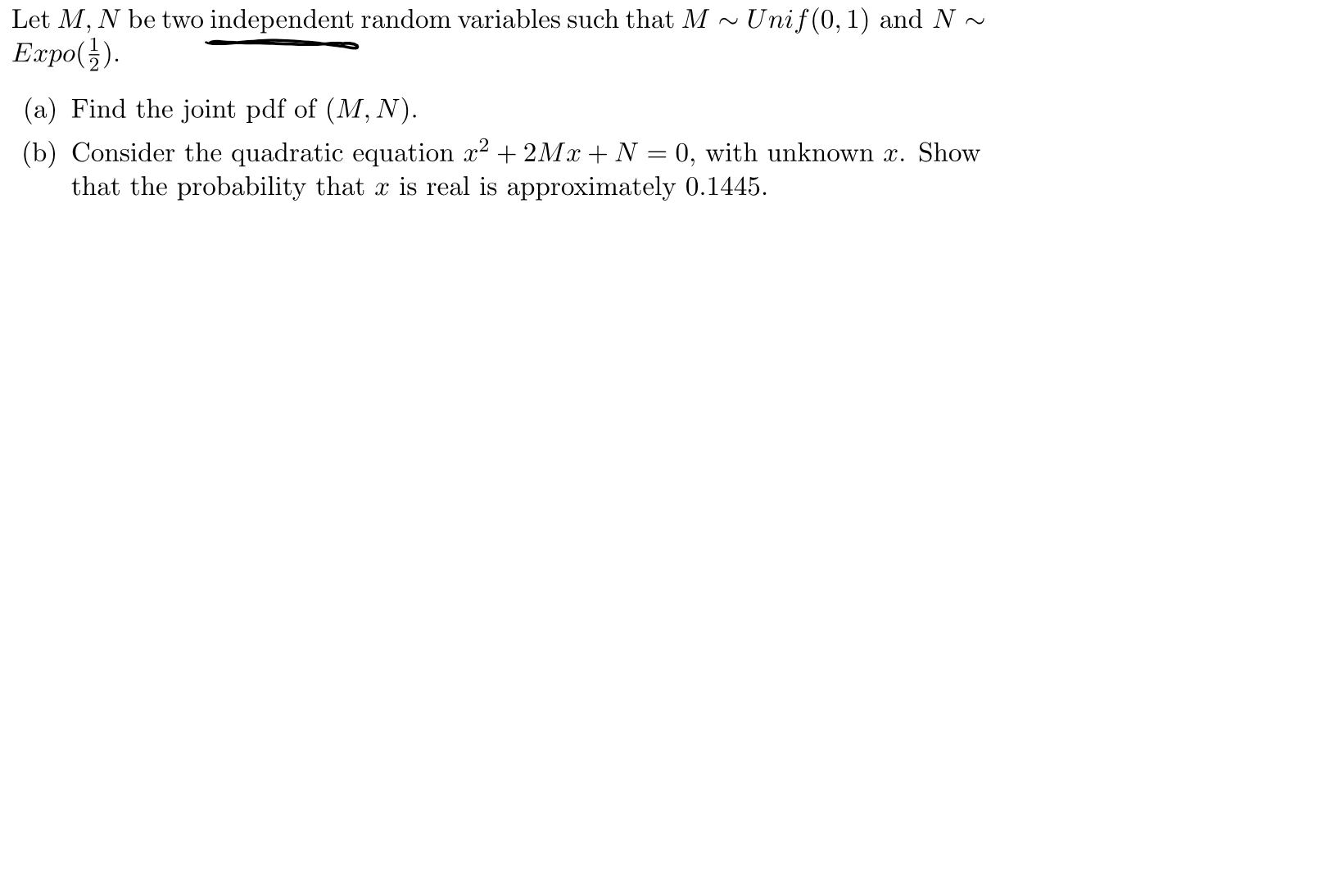# Let M, N be two independent random variables such that M~Unif(0,1) and N~Expo()(a) Find the joint pdf of (M, N).(b) Consider the quadratic equation 2 2MzN0, with unknown z. Showthat the probability that x is real is approximately 0.1445

Question
1 viewshelp_outlineImage TranscriptioncloseLet M, N be two independent random variables such that M~Unif(0,1) and N~ Expo() (a) Find the joint pdf of (M, N). (b) Consider the quadratic equation 2 2MzN0, with unknown z. Show that the probability that x is real is approximately 0.1445 fullscreen
check_circle

Step 1

Part (a):

Here, M ~ Unif (0, 1), N ~ Expo (1/2), which are independent. Thus, the joint pdf is:

Step 2

Part (b):

Step 3

Distribution of M2:

...

### Want to see the full answer?

See Solution

#### Want to see this answer and more?

Solutions are written by subject experts who are available 24/7. Questions are typically answered within 1 hour.*

See Solution
*Response times may vary by subject and question.
Tagged in

### Statistics